# Logical Reasoning for Bank and other Government exams - Set 3

In a certain code language, ‘sea is deep’ means ‘213’, ‘sky is blue’ means ‘514’ and ‘sea looks blue’ means ‘264’

What number is the code for ‘sea’?

a. 1
b. 2
c. 3
d. 4
e. 5
 Answer  Explanation ANSWER: 2 Explanation: In the first and second statements, the common digit is ‘1’ and the common word is ‘is’.So, ‘1’ is the code for ‘is’.In the second and third statements, the common digit is ‘4’ and the common word is ‘blue’.So, ‘4’ is the code for ‘blue’.Thus, in the second statement, ‘5’ is the code for ‘sky’.In the first and third statements, the common digit is ‘2’ and the common word is ‘sea’.So, ‘2’ is the code for ‘sea’.Thus, in the first statement, ‘3’ is the code for ‘deep’.In the third statement, ‘6’ is the code for ‘looks’.

2)   In the following question, choose the correct code form.

In a code language, ‘mok dan sil’ means ‘big bad wrestler’, ‘fit kon dan’ means ‘wrestler is good’, ‘cold tir fit’ means ‘he is new’.

Which word stands for ‘is’ in that language?

a. Fit
b. Kon
c. Dan
d. Mok
 Answer  Explanation ANSWER: Fit Explanation: In the first and second statements, the common code is ‘dan’ and the common word is ‘wrestler’. In the second and third statements, the common words is ‘fit’ and the common word is ‘is’.So, ‘fit’ means ‘is’.

• From a group of N contenders, a party has to select a leader.
• Even after holding a series of meetings, the politicians and the general body failed to reach a consensus.
• It was then proposed that all N contenders be given a number from 1 to N. Then they will be asked to stand on a podium in a circular arrangement, and counting would start from the contender numbered 1.
• The counting would be done in a clockwise fashion.
• The rule is that every alternate contender would be asked to step down as the counting continued, with the circle getting smaller and smaller, till only one person remains standing. Therefore, the first person to be eliminated would be the contender number 2.
If N is 18, which position should a contender choose if he has to be the leader?

a. 3
b. 5
c. 11
d. 17
 Answer  Explanation ANSWER: 5 Explanation: In this question, it would be about identifying a pattern. If you start small, you will find this pattern:When N is a power of 2, the number of the leader that gets selected is 1 and after that it moves in a sequence of odd numbers till the next power hits.Once you get the sequence, you would be able to solve the question. We need to find for N = 18.Nearest power of 2 is 16, for which the number of leader would be 1.Every subsequent jump of 1 in N will result in a jump of 2 in number of leader.Jumps in N = 18 - 16 = 2Jump in number of Leader = 2 x 2 = 4So, the number of leader who will get selected is 1 + 4 = 5.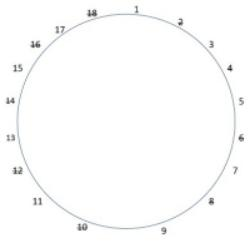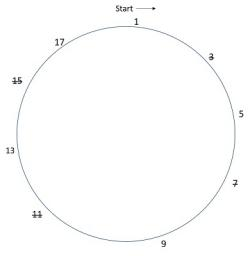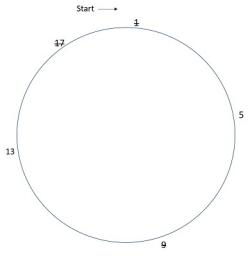After 3 rounds 5 and 13 will remain, beginning with 5, 13 will have to step down.Thus, 5 will be the leader.

4)   Choose the odd pair of words

a. Aphid – Paper
b. Termite - Wood
c. Moth - Wool
d. Locust – Plant
 Answer  Explanation ANSWER: Aphid – Paper Explanation: In all other pairs, first is the insect which damages the second.

5)   Choose the number which is different from others in the group

a. 6788
b. 6878
c. 6482
d. 9848
 Answer  Explanation ANSWER: 6482 Explanation: In all other numbers, sum of digits is 29, while in option C, it is 20.6 + 4 + 8 + 2 = 206 + 7 + 8 + 8 = 296 + 8 + 7 + 8 = 299 + 8 + 4 + 8 = 29

1. Rage, Raider, Rattler, Razor, Reaper, Rictor and Rogue are sitting in a circle facing at the centre.
2. Reaper is the neighbour Rage and Razor.
3. Rogue is not between Rictor and Rattler.
4. Rictor is to the immediate right of Rage.

Which of the following persons are sitting adjacent to each other from left to right in the order shown?

a. Raider, Rogue, Rattler
b. Rictor, Raider, Rattler
c. Rattler, Razor, Rogue
d. Reaper, Razor, Rogue
 Answer  Explanation ANSWER: Rictor, Raider, Rattler Explanation: Rictor is to immediate right of Rage.Reaper is the neighbor of Rage and Razor i.e. Reaper is between Rage and RazorRogue is not between Rictor and Rattler. So, Raider is between Rictor and Rattler.Thus, the arrangement is as shown -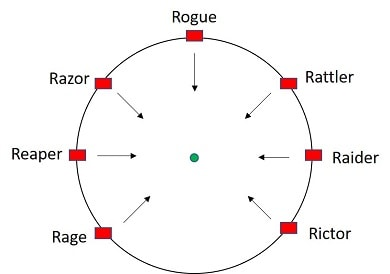The required group must contain three persons in consecutive positions in anticlockwise order. Such a group is Rictor, Raider, Rattler.

• Six people Nitro, Nemesis, Nick Fury, Viper, Kronos and Hulk are attending a hexagonal table conference.
• All the sides of the hexagon table so formed are of same length.
• Nitro is not adjacent to Nemesis or Nick Fury, Viper is not adjacent to Nick Fury or Kronos.
• Nemesis and Nick Fury are adjacent, Hulk is in the middle of Viper and Nick Fury.
Which of the following pair is neighbor pair of Hulk?

a. Nitro and Viper
b. Viper and Nemesis
c. Nemesis and Kronos
d. Nick Fury and Viper
 Answer  Explanation ANSWER: Nick Fury and Viper Explanation: Hulk is in the middle of Viper and Nick Fury.Nemesis and Nick Fury are adjacent.Nitro is not adjacent to Nick Fury.Thus, two arrangements are possible.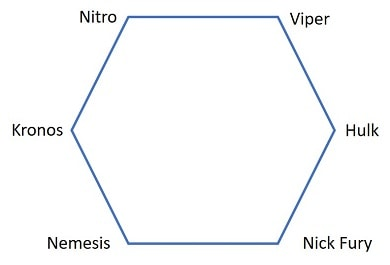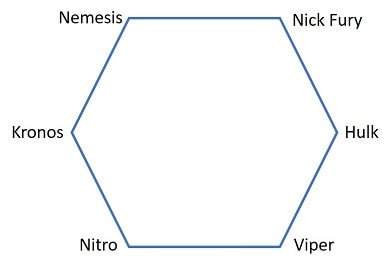Viper and Nick Fury are neighbors of the Hulk.

8)   In the following question, one term in the number series is wrong. Find the wrong term.

3, 8, 15, 24, 35, 49, 63

a. 15
b. 35
c. 49
d. 63
 Answer  Explanation ANSWER: 49 Explanation: The difference between consecutive terms in the above series are 5, 7, 9, 11, 13, 15Here, 49 is the wrong number.It should be replaced by (35 + 13) = 48

9)   In the following question, various terms of an alphabet series are given with one or more missing terms as shown by (?). Choose the missing term out of the given alternatives.

R, U, X, ?, D

a. Z
b. B
c. A
d. Y
 Answer  Explanation ANSWER: A Explanation: The pattern in this series is +3R __+3__ U __+3__ X __+3__ A __+3__ DThe missing alphabet should be A

10)   In the question below is given a statement followed by two courses of action numbered I, II and III. You have to assume everything in the statement to be true and based on the information given in the statement, decide which of the suggested courses of action logically follow(s) for pursuing.

(A) If only I follows
(B) If only II follows
(C) If either I or II follows
(D) If neither I nor II follow
(E) If both I and II follow.

Statement - While crossing a bridge, a train derailed and fell into the swelling waters of River Yamuna, causing death of more than 50 people.

Courses of Action - I. The Authorities should blame the Government for the accident.

II. The Government should allocate funds to compensate the victims and recover from destruction caused.

III. To avoid accidents in future, the protection walls of the bridge should be made stronger.

a. Only I follows
b. Only II follows
c. Only II and III follow
d. Neither I nor II follows
e. Both I and II follow
 Answer  Explanation ANSWER: Only II and III follow Explanation: The necessary thing here is to take preventive measures to protect the passengers to avoid re-occurrence of such events. So, III follows here.And, the Government should pay the victims and their families adequate compensation. Hence, II follows.Here, II and III both follow.

11)   In the question below is given a statement followed by two courses of action numbered I and II. You have to assume everything in the statement to be true and based on the information given in the statement, decide which of the suggested courses of action logically follow(s) for pursuing.

(A) If only I follows
(B) If only II follows
(C) If either I or II follows
(D) If neither I nor II follow
(E) If both I and II follow.

Statement - It is estimated that about thirty lakh tourists will visit the city during winter season.

Courses of Action - I. The civic authority should monitor the crowd and restrict entry of the people.

II. The local police authority should to maintain law and order during the season.

III. Hospitals in the city should be put on high alert in case of any eventuality.

a. Only I follows
b. Only II follows
c. Only I and II follows
d. Neither I nor II follows
e. Both II and III follow
 Answer  Explanation ANSWER: Both II and III follow Explanation: Tourists cannot be deprived from enjoying the season, so I cannot be followed.Further, it may prove useful to put hospitals on alert to be ready to provide quick medical aid to patients in case of any eventuality and similarly, the police should also be alert.So, both II and II follow.

12)   In the following question, a statement/group of statements is given followed by some conclusions. Without resolving anything yourself, choose the conclusion which logically follows from the statement(s).

Statement - All good books help and so will this one.

a. This is not the best book.
c. This is a good book.
d. Some good books do not help.
 Answer  Explanation ANSWER: This is a good book. Explanation: No explanation is available for this question!

13)   In the following question, a statement/group of statements is given followed by some conclusions. Without resolving anything yourself, choose the conclusion which logically follows from the statement(s).

Statement - One must work hard to score well in the examination.

a. Examination is not related with hard work.
b. All those who work hard, never score good.
c. Hard-working person is a stupid person.
d. Without hard-work one is bound to fail.
 Answer  Explanation ANSWER: Without hard-work one is bound to fail. Explanation: No explanation is available for this question!

14)   If you are facing north-east and move 12 m forward, turn left and move 5 m, then you are -

a. East of your initial position
b. West of your initial position
c. South of your initial position
d. North of your initial position
 Answer  Explanation ANSWER: North of your initial position Explanation: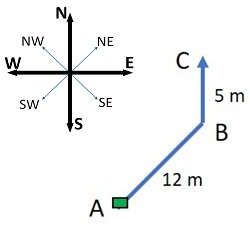15)   Ravindra Jadeja left home and ran 20 km towards South, then turned right and ran 10 km.
He took rest for ten minutes at the same spot and then again turned right and ran 20 km.
After this he turned left and ran 20 km to the cricket ground.

How many kilometers will he have to run back to reach his home?

a. 15 km
b. 20 km
c. 25 km
d. 30 km
 Answer  Explanation ANSWER: 30 km Explanation: Required distance AE = 10 + 20 = 30 km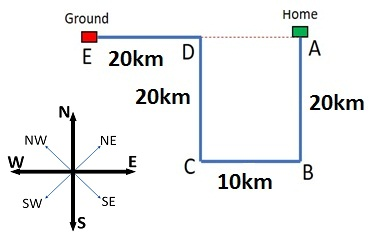1. M \$ N means M is the mother of N
2. M # N means M is the father of N
3. M @ N means M is the husband of N
4. M % N means M is the daughter of N

Which of the following expressions indicate ‘R is the sister of H’?

a. H \$ D @ F # R
b. R % D @ F \$ H
c. R \$ D @ F # H
d. None of these
 Answer  Explanation ANSWER: R % D @ F \$ H Explanation: R % D @ F \$ H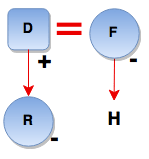1. M \$ N means M is the mother of N
2. M # N means M is the father of N
3. M @ N means M is the husband of N
4. M % N means M is the daughter of N

Which of the following expressions indicate ‘H is the brother of N’?

a. H # R \$ D \$ N
b. N % F @ D \$ H
c. N % F @ D % H
d. N % F @ D \$ H # R
 Answer  Explanation ANSWER: N % F @ D \$ H # R Explanation: N % F @ D \$ H # R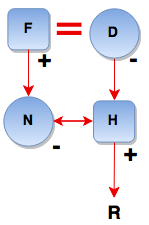18)   At what time between 3 & 4 will the hands of a watch point in opposite directions?

a. 43 min past 3
b. 42 (5/11) min past 3
c. 49 (1/11) min past 3
d. 50 min past 3
 Answer  Explanation ANSWER: 49 (1/11) min past 3 Explanation: At 3 o'clock, the hands of the watch are 15 min. space apart.To be in opposite directions, they must be 30 min. spaces apart. Minute hand must gain 45 min. space (15 + 30 min)55 min spaces are gained in 60 min. 45 min. spaces would be gained in (60/55 × 45) min. Required time = 49 1/11 min. past 3.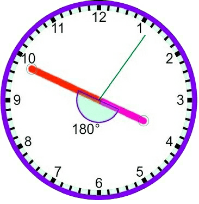19)   In the question below is given a statement followed by two conclusions numbered I and II.

(A) If only I follows
(B) If only II follows
(C) If either I or II follows
(D) If neither I nor II follows
(E) If both I and II follow.

Statement - I. All balls are baskets.

Conclusion - I.  All balls are tubs.
II. All tubs are balls.

a. Only I follows
b. Only II follows
c. Either I or II follows
d. Neither I nor II follows
e. Both I and II follow
 Answer  Explanation ANSWER: Only I follows Explanation: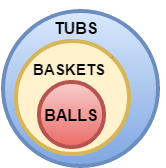20)   In given below has a set of three or four statements. Each set of statements is further divided into three segments. Choose the alternative where the third segment in the statement can be logically deduced using both the preceding two, but not just from one of them.

Statement - A. Many writers are not readers. All plumbers are writers. Some plumbers are not readers.

B. Boys play cricket. Some girls do not play cricket. Some girls are not boys.

C. All Humans live in houses. Some penguins live in houses. Some penguins are Humans.

a. A
b. B
c. C
d. B and C
 Answer  Explanation ANSWER: B Explanation: X – indicates there is no relation between the given elements.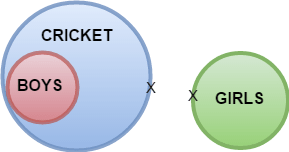21)   The following questions consists of figures marked A, B, C, D called the Problem Figures followed by other figures marked 1, 2, 3, 4 called the Answer Figures.

Select a figure from amongst the Answer Figures which will continue the same series as established by the Problem Figures.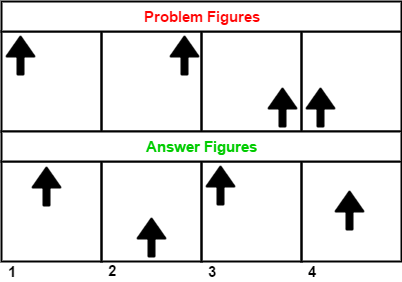a. 1
b. 2
c. 3
d. 4
 Answer  Explanation ANSWER: 3 Explanation: The element is moving from corner to corner in a clockwise order. The answer figure should be figure 3

22)   In the following question consists of figures marked A, B, C, D called the Problem Figures followed by other figures marked 1, 2, 3, 4 called the Answer Figures.

Select a figure from amongst the Answer Figures which will continue the same series as established by the Problem Figures.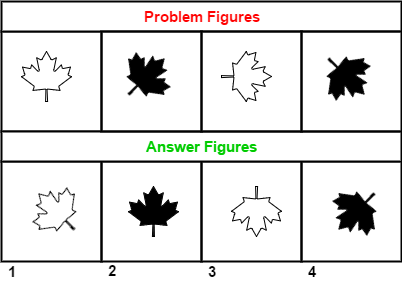a. 1
b. 2
c. 3
d. 4
 Answer  Explanation ANSWER: 3 Explanation: The leaf is rotated through 45 degrees in clockwise order with alternating shallow and filled leaf. The answer figure should be figure 3.